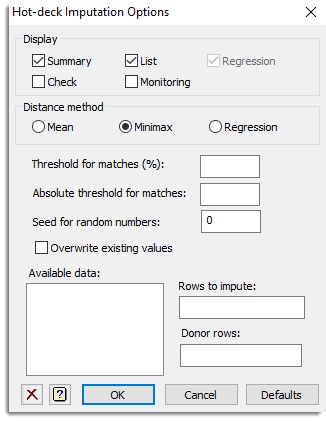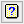1. Home
2. Hot-deck Imputation Options

# Hot-deck Imputation Options

Use this to select options and output to be used by hot-deck imputation for surveys data.## Display

Specifies which items of output are to be displayed in the Output window.

 Summary A summary of the imputation Check Displays correlations as well as a scatter plot of the predictions against the actual data List A list of the recipients and donors Monitoring Provides information about each match Regression Displays details of the regression model when the regression distance method is used

## Distance method

Specifies the method used for calculating distances. The Minimax setting uses an approach where the best match is the one with the minimum value of the maximum absolute difference between any of the distance variables (specified on the main menu). The Mean option uses the mean of the absolute differences and the Regression setting calculates distances on the basis of predictions from a regression model.

## Threshold for matches (%)

By default, where possible the single best match for each unit is determined. In many cases it is required to select one at random from the closest matches. This option can be used to specify a tolerance to use in these situations. For example, setting this value to 10 will request that the match is selected at random from amongst the donors with distance up to 10% greater than the minimum distance.

## Absolute threshold for matches

Specifies the distance relative to the minimum in absolute terms. Usually you would set this to a value relative to the minimum distance, however you can set this to a negative value. If a negative value is supplied this is taken to mean that a match is selected at random from those with a distance less than the absolute value that is supplied. For example, if this is set to -0.2 and the mean distance method is selected, any units with a mean distance of less 0.2 from the unit to be imputed are considered matches, and one of these is selected at random. Alternatively, if this is set to 0.2 and the best match is for example 0.18, any units with a mean distance of less than 0.18 + 0.2 = 0.38 are considered matches, and one of these is selected at random.

## Seed for random numbers

Specifies the seed used to generate the random numbers. The default value of zero initializes the seed at random if this is the first time that the Genstat randomization routines have been used in the current job; otherwise it continues the existing sequence of random numbers.

## Overwrite existing values

When selected, imputed values will always be inserted. Alternatively when this option is not selected imputed values will only be used to replace those that are missing.

## Rows to impute

Specifies a variate containing logical (0 or 1) values to indicate whether each unit is to be imputed. Alternatively a value can be used to specify a number of rows to be selected at random to be imputed to allow the effectiveness of the imputation process to be studied.

## Donor rows

Specifies a variate containing logical (0 or 1) values indicating whether each unit can be used as a donor.

## Action buttons

 OK Save the option settings and close the dialog. Cancel Close the dialog without making any changes. Defaults Resets the options to their default settings.

## Action IconsClear Clear all fields and list boxes.Help Open the Help topic for this dialog.